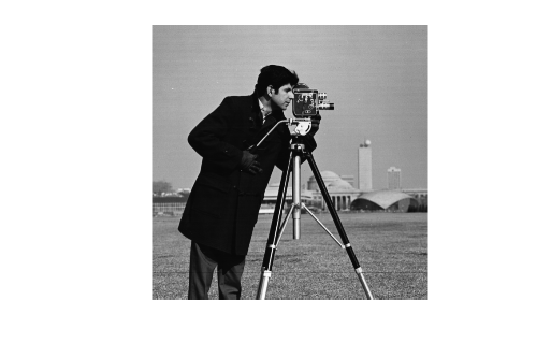# createUMat

Create MATLAB interface object for OpenCV `UMat` class

## Syntax

``[ocvUMat,ocvInputArray] = createUMat(img)``
``[ocvUMat,ocvArray] = createUMat(img,arrayType)``
``[ocvUMat,ocvOutputArray] = createUMat``
``[ocvUMat,ocvArray] = createUMat(arrayType)``

## Description

example

````[ocvUMat,ocvInputArray] = createUMat(img)` creates the MATLAB® interface objects for the OpenCV `UMat` class and the associated `InputArray` class. Use these interface objects to pass an image as input to the OpenCV functions.```

example

````[ocvUMat,ocvArray] = createUMat(img,arrayType)` creates the MATLAB interface objects for the OpenCV `UMat` class and the associated `InputArray`, `OutputArray`, or``` InputOutputArray``` class specified by `arrayType`.```

example

````[ocvUMat,ocvOutputArray] = createUMat` creates an empty interface object for the OpenCV `UMat` class and the associated `OutputArray` class. Use this interface to write the output returned by an OpenCV function.```
````[ocvUMat,ocvArray] = createUMat(arrayType)` creates an empty interface object for the OpenCV `UMat` class and the associated `InputArray`, `OutputArray`, or `InputOutputArray` class specified by `arrayType`.```

## Examples

collapse all

Add the prebuilt MATLAB interface to OpenCV package name to the import list.

`import vision.opencv.util.*`

Create MATLAB Interface Object for OpenCV `UMat` and `InputArray` Class

Read an image into the MATLAB workspace.

`img = imread("cameraman.tif");`

Create a MATLAB interface object for the OpenCV `UMat` class and store the image data. The function also creates an interface object for the OpenCV `InputArray` class that is constructed from the `UMat` class.

`[ocvMat,ocvArray] = createUMat(img);`

Display and inspect the properties of the MATLAB interface object for the OpenCV `UMat` class.

`ocvMat`
```ocvMat = UMat with properties: flags: 1124024320 dims: 2 rows: 256 cols: 256 allocator: [1x1 clib.opencv.cv.MatAllocator] usageFlags: USAGE_DEFAULT u: [1x1 clib.opencv.cv.UMatData] offset: 0 size: [1x1 clib.opencv.cv.MatSize] step: [1x1 clib.opencv.cv.MatStep] ```

Inspect the type of array class returned by the function.

`ocvArray`
```ocvArray = x_InputArray with no properties. ```

Use the `getImage` utility function to read the image stored in the `InputArray` class. Display the image.

```outImg = getImage(ocvArray); figure imshow(outImg)```Create MATLAB Interface Object for OpenCV `UMat` and `OutputArray` Classes

Add the prebuilt MATLAB interface to OpenCV package name to the import list.

`import vision.opencv.util.*`

Create a MATLAB interface object for the OpenCV `UMat` class. The function also creates an interface object for the OpenCV `OutputArray` class that is constructed from the `UMat` class.

`[ocvMat,ocvArray] = createUMat;`

Display and inspect the properties of the MATLAB interface object for the OpenCV `UMat` class.

`ocvMat`
```ocvMat = UMat with properties: flags: 1124007936 dims: 0 rows: 0 cols: 0 allocator: [1x1 clib.opencv.cv.MatAllocator] usageFlags: USAGE_DEFAULT u: [1x1 clib.opencv.cv.UMatData] offset: 0 size: [1x1 clib.opencv.cv.MatSize] step: [1x1 clib.opencv.cv.MatStep] ```

Inspect the type of array class returned by the function.

`ocvArray`
```ocvArray = x_OutputArray with no properties. ```

Specify Array Class to Construct from OpenCV `UMat` Class

Add the prebuilt MATLAB interface to OpenCV package name to the import list.

`import vision.opencv.util.*`

Read an image into the MATLAB workspace.

`img = imread("cameraman.tif");`

Create a MATLAB interface object for the OpenCV `UMat` class. Specify the array type as "`InputOutput"` for the function to construct the OpenCV `InputOutputArray` class from the `UMat` class.

```arrayType = "InputOutput"; [ocvMat,ocvArray] = createUMat(img,arrayType);```

Display and inspect the properties of the MATLAB interface object for the OpenCV `UMat` class.

`ocvMat`
```ocvMat = UMat with properties: flags: 1124024320 dims: 2 rows: 256 cols: 256 allocator: [1x1 clib.opencv.cv.MatAllocator] usageFlags: USAGE_DEFAULT u: [1x1 clib.opencv.cv.UMatData] offset: 0 size: [1x1 clib.opencv.cv.MatSize] step: [1x1 clib.opencv.cv.MatStep] ```

Inspect the type of array class returned by the function.

`ocvArray`
```ocvArray = x_InputOutputArray with no properties. ```

## Input Arguments

collapse all

Input image, specified as an M-by-N matrix representing an intensity image or M-by-N-by-3 array representing a color image.

Data Types: `single` | `double` | `int8` | `int16` | `int32` | `uint8` | `uint16`

Type of array to associate with the MATLAB interface object for OpenCV `UMat` class, specified as : `"Input"`, `"Output"`, or `"InputOutput"`.

Data Types: `char` | `string`

## Output Arguments

collapse all

Unified n-dimensional dense array class, returned as a `UMat` interface object. This MATLAB interface object is a representation of the OpenCV class `cv::UMat`.

Proxy class for passing inputs to OpenCV functions, returned as an `InputArray` interface object. This MATLAB interface object is a representation of the OpenCV class `cv::_InputArray`.

Proxy class for inputs or outputs, returned as one of these values:

• `InputArray` interface object — This MATLAB interface object is a representation of the OpenCV class `cv::_InputArray`. This value is returned if `arrayType` is specified as `"Input"`.

• `InputOutputArray` interface object — This MATLAB interface object is a representation of the OpenCV class, `cv::_InputOutputArray`. This value is returned if `arrayType` is specified as `"InputOutput"`.

• `OutputArray` interface object — This MATLAB interface object is a representation of the OpenCV class `cv::_OutputArray`. This value is returned if `arrayType` is specified as `"Output"`.

Proxy class for writing outputs from OpenCV functions, returned as an `OutputArray` interface object. This MATLAB interface object is a representation of the OpenCV class `cv::_OutputArray`.

## Version History

Introduced in R2021b